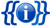Documentation The documentation below is transcluded from Template:IsNumber/doc. (edit | history)

This template is used to determine whether an input is an integer number. This is useful in cases where it is ambiguous if, for instance, the value of a template parameter is the name or the ID of an item.

It only recognizes purely numerical strings, i.e., strings consisting of one or more digits. It also recognizes a minus sign in front of the digits (if the `\$onlypositive` parameter is not set). Spaces within the number, or thousands or decimal separators are not considered (spaces around the number are ignored).

Note that it might sometimes be preferable to use a combination of `{{#iferror:}}` and `{{#expr:}}`. That allows recognizing all valid `{{#expr:}}` syntax, e.g. formulas, which might be useful if the following logic uses `{{#expr:}}` itself.

## Usage

`{{ isNumber | <string to check> | onlypositive= y/yes }}`

First unnamed parameter

The string which is to be checked if it consists of a number.

onlypositive

Set this parameter to disregard numbers with a minus sign in the front.

## Examples

Code Result
`{{isNumber|42}}` y
`{{isNumber|forty-two}}`
`{{isNumber| 42 }}` y
`{{isNumber|4 2}}`
`{{isNumber|-42}}` y
`{{isNumber|-42|onlypositive=y}}`
`{{isNumber|- 42}}`
`{{isNumber|42.1}}`
`{{isNumber|42,000}}`
`{{isNumber|42000}}` y
`{{isNumber|}}`
`{{isNumber|-}}`